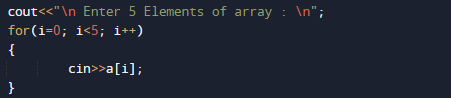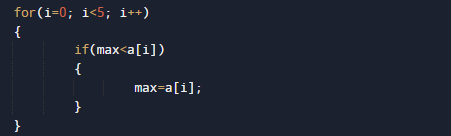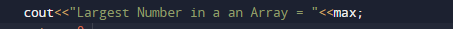# C++ Program to Find Largest Element of an Array

In this tutorial you will learn about the C++ Program to Find Largest Element of an Array and its application with practical example.

In this tutorial, we will learn to create a C++ program that will Find Largest  Elements of an Array using C++ programming.

## Prerequisites

Before starting with this tutorial we assume that you are best aware of the following C++ programming topics:

• Operators.
• looping statements.
• Basic input/output.
• Basic c++ programming
• For Loop.
• Array.

## What is array?

An Array is a collection variable elements  that can store multiple values in a single variable of same type.In Computer Science,an array is a data structure, or simply an array, is a data structure consisting of a collection of elements.

## C++ Program to Find Largest Element of an Array

In this program we will find Largest Element in an Array. We would first declared and initialized the required variables. then we would prompt user to input elements of array .Later we will find the Biggest element in an Array.

## OutputIn the above program, we have first declared and initialized a set variables required in the program.

• a = it will hold entered elements.
• max =it will give largest number in an array.
• i = for iteration.

In our program, we will take 5 values form user in an array ,after taking these values we will find the maximum number in  an array.

First of all we will take 5 values from user  in variable a.After that we will initialize max=a i.e first element of a an array.Now we compare the value of max with each and every value present in array a. While comparing, if the value of max  is less than in condition, then the value of max  (value which is greater than max values) swapped and gets initialized to max and at the end we will get the maximum value within the  array in max.In this tutorial we have learn about the C++ Program to Find Largest Element of an Array and its application with practical example. I hope you will like this tutorial.